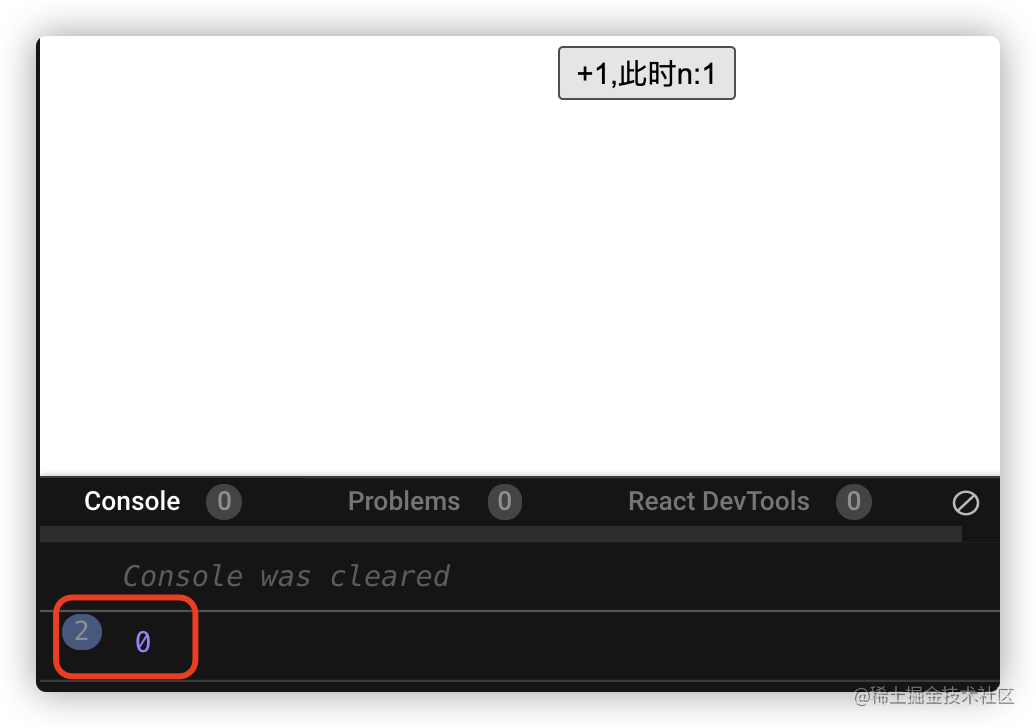Hooks系列主要分以下内容：

# 从简单示例开始

``````export default function App() {
const initValue = 0;
const [n, setN] = useState(initValue);
const add = () => {
setN(n + 1);
};
return (
<div className="App">
</div>
);
}

## useState用法

const [state, setState] = useState(initValue)

useState接收一个初始值，这个初始值可以是对象，也可以是简单数据类型。

## 规则

useState不能在内部的循环、条件判断、嵌套的方法中使用。

## setState异步更新

``````export default function App() {
const initValue = 0;
const [n, setN] = useState(initValue);
const add = () => {
setN(n + 1);
+  console.log(n)
+  setN(n+1)
+  console.log(n)
};
return (
<div className="App">
</div>
);
}## setN传函数

setN是可以传递函数的，它的参数是旧的n，返回新的value

``````export default function App() {
const initValue = 0;
const [n, setN] = useState(initValue);
const add = () => {
+   setN((oldN) => {
+   	console.log(oldN);
+   	return oldN + 1;
});
+  setN((oldN) => {
+ 	console.log(oldN);
+  	return oldN + 1;
});
};
return (
<div className="App">
</div>
);
}

# state可以是对象

## 错误示例

``````export default function App() {
const initValue = { n: 0, m: 0 };
const [state, setState] = useState(initValue);
const addN = () => {
setState((state) => {
return { n: state.n + 1 };
});
};
const addM = () => {
setState((state) => {
return { m: state.m + 1 };
});
};
return (
<div className="App">
</div>
);
}

## 正确示例

``````export default function App() {
const initValue = { n: 0, m: 0 };
const [state, setState] = useState(initValue);
const addN = () => {
setState((state) => {
+  return { ...state, n: state.n + 1 };
});
};
const addM = () => {
setState((state) => {
+  return { ...state, m: state.m + 1 };
});
};
return (
<div className="App">
</div>
);
}

# 总结

• useState返回一对数组，第一个值是初始值，第二个值state的setter方法
• 如果state是对象的话，需要我们手动合并
• setState时，可以传函数也可以传值，如果依赖于旧的state，最好使用函数的形式

CS_Joe 掘金分享